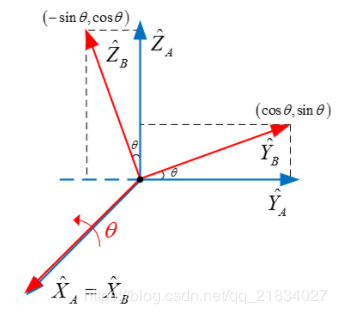# 2.1位置和姿态的表示

$^AP=\begin{bmatrix} p_{x} \\ p_{y} \\ p_{z} \end{bmatrix} \tag{2.1} ​$

$^A_{B}R=\begin{bmatrix} ^A{x_{B}} & ^A{y_{B}} & ^A{z_{B}} \end{bmatrix} = \begin{bmatrix} r_{11} & r_{12} & r_{13} \\ r_{21} & r_{22} & r_{23} \\ r_{31} & r_{23} & r_{33} \end{bmatrix} \tag {2.2}$ $^A_{B}R$称为旋转矩阵$^A_{B}R$记作(矩阵｛B｝相对于矩阵｛A｝的表达)，且满足条件 $^A_{B}R^{-1} = {^A_{B}R^T}$$\left| ^A_{B}R\right| =1$

$^A_{B}R = \begin{bmatrix} ^A{x_{B}} & ^A{y_{B}} & ^A{z_{B}} \end{bmatrix} = \begin{bmatrix} X_B⋅X_A & Y_B⋅X_A & Z_B⋅X_A \\ X_B⋅Y_A & Y_B⋅Y_A & Z_B⋅Y_A \\ X_B⋅Z_A & Y_B⋅Z_A & Z_B⋅Z_A\end{bmatrix} \tag {2.3}$

$R\left( x,\theta \right) = \begin{bmatrix} 1 & 0 & 0 \\ 0 & c\theta & -s\theta \\ 0 & s\theta & c\theta \end{bmatrix} \tag {2.4}$
$R\left( y,\theta \right) = \begin{bmatrix} c\theta & 0 & s\theta \\ 0 & 1 & 0 \\ -s\theta & 0 & c\theta \end{bmatrix} \tag {2.5}$
$R\left( z,\theta \right) = \begin{bmatrix} c\theta & -s\theta & 0 \\ s\theta & c\theta & 0 \\ 0 & 0 & 1 \end{bmatrix} \tag {2.6}$
s表示sin，c表示cos

（1）${^AX_B} ⋅ {^AX_B} = {^AY_B} ⋅ {^AY_B} = {^AZ_B} ⋅ {^AZ_B} = 1$
（2）${^AX_B} ⋅ {^AY_B} = {^AY_B} ⋅ {^AZ_B} = {^AZ_B} ⋅ {^AX_B} = 0$$R\left( x,\theta \right) = \begin{bmatrix} 1 & 0 & 0 \\ 0 & c\theta & -s\theta \\ 0 & s\theta & c\theta \end{bmatrix} \tag {2.7}$

${B}=\left\{{^A_B}R , \ ^AP_{B_o}\right\} { }\tag {2.8}$ 当表示位置时，式(2.8)中的旋转矩阵 ${^A_B}R=I$ (单位矩阵)；当表示姿态时，式(2.8)中位置矢量 $^AP_{B_o} = o$

©️2019 CSDN 皮肤主题: 技术工厂 设计师: CSDN官方博客